# Test: Probability & Statistics

## 20 Questions MCQ Test RRB JE for Electrical Engineering | Test: Probability & Statistics

Description
Attempt Test: Probability & Statistics | 20 questions in 60 minutes | Mock test for GATE preparation | Free important questions MCQ to study RRB JE for Electrical Engineering for GATE Exam | Download free PDF with solutions
QUESTION: 1

### If the arithmetic mean of two numbers is 10 and their geometric mean is 8, the numbers are

Solution:

Let the numbers be a and b Then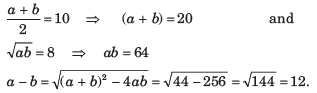Solving a + b = 20 and a - b = 12 we get a  = 16 and b = 4.

QUESTION: 2

### The median of 0, 2, 2, 2, -3, 5, -1, 5, 5, -3, 6, 6, 5, 6 is

Solution:

Observations in ascending order are -3, -3, -1, 0, 2, 2, 2, 5, 5, 5, 5 6, 6, 6
Number of observations is 14, which is even.QUESTION: 3

### Consider the following table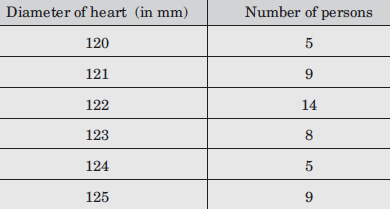The median of the above frequency distribution is

Solution:

The given Table may be presented as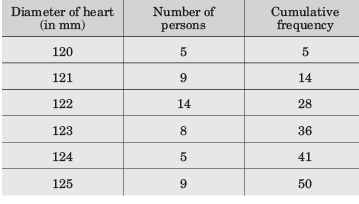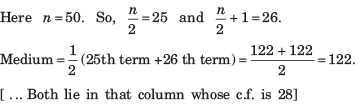QUESTION: 4

The mode of the following frequency distribution, is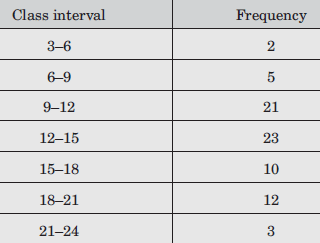Solution:

Maximum frequency is 23. So, modal class is 12–15.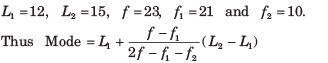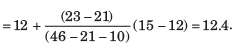QUESTION: 5

The mean-deviation of the data 3, 5, 6, 7, 8, 10, 11, 14 is

Solution: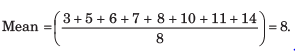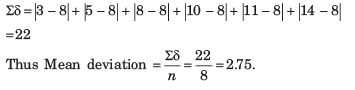QUESTION: 6

The mean deviation of the following distribution is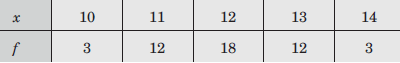Solution: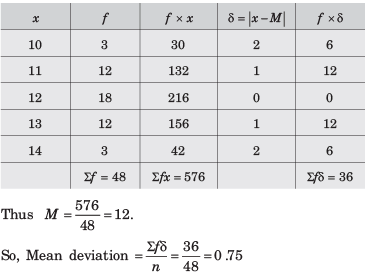QUESTION: 7

The standard deviation for the data 7, 9, 11, 13, 15 is

Solution: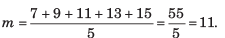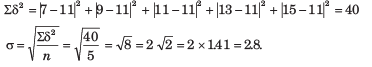QUESTION: 8

The standard deviation of 6, 8, 10, 12, 14 is

Solution: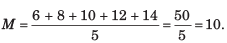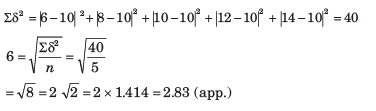QUESTION: 9

The probability that an event A occurs in one trial of an experiment is 0.4. Three independent trials of experiment are performed. The probability that A occurs at least once is

Solution:

Here p  = 0.4, q = 0.6 and n = 3.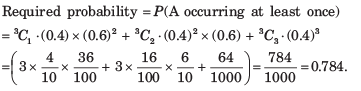QUESTION: 10

Eight coins are tossed simultaneously. The probability of getting at least 6 heads is

Solution:

p = 1/2, q = 1/2, n = 8. Required probability  = P (6 heads or 7 heads or 8 heads)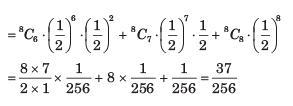QUESTION: 11

The ranks obtained by 10 students in Mathematics and Physics in a class test are as follows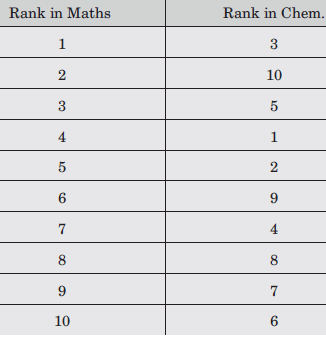The coefficient of correlation between their ranks is

Solution:

Di = -2, -8, -2, 3, 3, -3, 3, 0, 2, 4.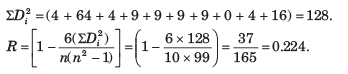QUESTION: 12

If ∑ xi = 24, ∑yi = 44, ∑ xiyi =  306,  ∑ xi2 =164 ,∑yi2 = 574 and n = 4 then the regression coefficient bxy  is equal to

Solution: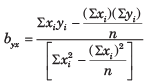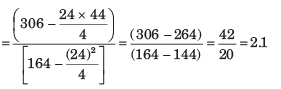QUESTION: 13

If ∑ xi = 30, ∑yi = 42, ∑ xiyi =  199 , ∑ xi2 =184 ,∑yi2 = 318 and n = 6 then the regression coefficient bxy  is equal to

Solution: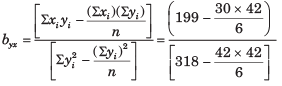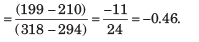QUESTION: 14

Let r be the correlation coefficient between x and y and bxy ,byx be the regression coefficients of y on x and x on y respectively then

Solution: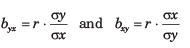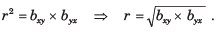QUESTION: 15

Which one of the following is a true statement

Solution: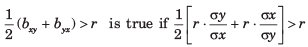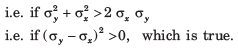QUESTION: 16

If byx  = 1.6 and bxy = 0.4 and θ is the angle between two regression lines, then tan θ is equal to

Solution: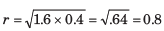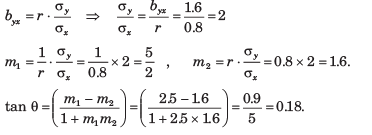QUESTION: 17

The equations of the two lines of regression are : 4x + 3y + 7 = 0 and 3x + 4y + 8  = 0. The correlation coefficient between x and y is

Solution: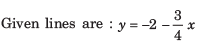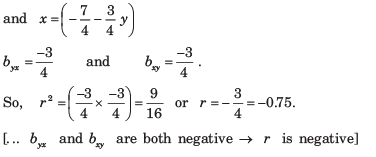QUESTION: 18

If cov(X, Y) = 10, var (X) = 6.25 and var(Y)  = 31.36, then ρ(X,Y) is

Solution: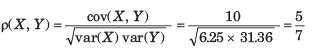QUESTION: 19

If ∑x = ∑y = 15, ∑x2 = ∑y2 = 49, ∑xy = 44 and n = 5, then bxy = ?

Solution: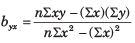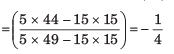QUESTION: 20

If ∑x = 125, ∑y = 100 , ∑x2 = 1650, ∑y2 = 1500 , ∑xy = 50 and n = 25, then the line of regression of x on y is

Solution: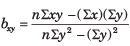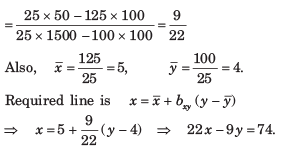Use Code STAYHOME200 and get INR 200 additional OFF Use Coupon Code